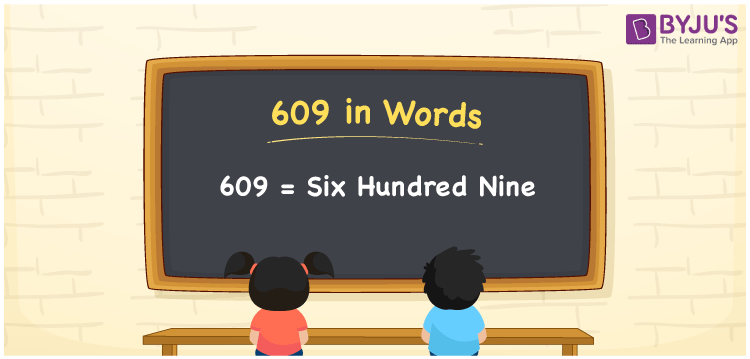# 609 in Words

The number 609 in words is “Six Hundred Nine”. As we know that the number 609 is a natural number and it has three digits. We generally use the place value system for converting 609 into words. Also, 609 is a cardinal number. For example, if you invested Rs. 609 on purchasing toys, you can write it as “ I invested Rs. Six hundred nine on purchasing toys”.

 609 in Words: Six hundred nine. Six hundred nine in Numerical Form: 609.

## 609 in English Words## How to Write 609 in Words?

The below table represents the place values of 609:

 Hundreds Tens Ones 6 0 9

The expanded form of 609 is as follows:

= 6 × Hundred + 0 × Ten + 9 × One

= 6 × 100 + 0 × 10 + 9 × 1

= 600 + 9

= 609

= Six hundred nine

Hence, 609 in words is six hundred nine.

The number 609 appears between 608 and 610.

609 in words – Six hundred nine

Is 609 an odd number? – Yes

Is 609 an even number? – No

Is 609 a perfect square number? – No

Is 609 a perfect cube number? – No

Is 609 a prime number? – No

Is 609 a composite number? – Yes

## Frequently Asked Questions on 609 in Words

Q1

### How to write 609 in words?

609 in words is six hundred nine.

Q2

### Simplify 600 + 9, and express it in words.

Simplifying 600 + 9, we get 609. Hence, 609 in words is six hundred nine.

Q3

### Is 609 a prime number?

No, 609 is not a prime number.